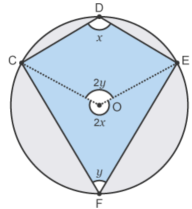# Circle theorems: cyclic quadrilateral opposite angles

1. Thales. The easiest circle theorem.
2. Inscribed angle. The angle subtending a major arc is constant.
3. Cyclic quadrilateral - opposite angles sum to 180°. Plus minor arc.
4. Tangent radius. Blindingly obvious, but a slippery proof.
5. Alternate segment. Perhaps the most unintuitive.
6. Chord bisection by radius. The relevant angle is 90°.
7. Equal length tangents. For completeness.

A cyclic quadrilateral is one whose four vertices can be placed on the circumference of a circle. Not all quadrilaterals are cyclic.

## Two results

1. Opposite angles of a cyclic quadrilateral sum to 180°.
2. On the minor arc ∠BQA is constant.

From the inscribed angle theorem the angle a chord makes with a point on its major arc is constant. Putting this together with (1) leads immediately to (2).

## Try it yourself

We’ve seen this diagram before - for the inscribed angle theorem, where the point Q is not usually drawn. But here it allows us to draw a cyclic quadrilateral.

In the diagram below you can move around any point except O.

Before we do this, let’s think about the set up, to ensure we don’t fall into a diagram dependency trap.

Four points on a circle might appear to be awkwardly placed: perhaps all fall one side of a diameter. But in any situation we can draw a line between the first and third points, A and B, rotating the diagram so that the AB chord is horizontal.

We now have P on the major arc and Q on the minor arc. We do not know the length of AB, the exact positioning of P or Q or the angles ∠APB or ∠AQB.

But just have a play. Move A and B around until you’re happy. Then keep A and B fixed and move P and/or Q. Note that:

1. ∠APB is constant, as expected from the inscribed angle theorem.
2. ∠APB + ∠AQB = 180°.
3. ∠AQB is constant.The proof at BBC bitesize - essentially summarized to the left - seems misleading. It assumes the constant angle on a minor arc result, but they have proved only the major arc result.

The problem is that the x and 2x parts have not been justified, nor is it obvious why we can assume that O is inside ΔCEF.

Our proof is quite different.

This is my own proof, though it would be a surprise if it existed nowhere else.

From the inscribed angle theorem we know that ∠APB is constant (= α say) as P varies over the major arc. For any given Q this means that ∠APB + ∠AQB is constant as P varies over the major arc.

This means that we are allowed to choose P conveniently. This is a rather neat reversal of my normal diagram dependency proof critique.

We choose P so that O lies within the ΔAPB. We draw additional radii OP and OQ. We then have four isosceles triangles. We set their equal angles α, β, γ, and δ as shown.The sum of the angles in the four triangles is also the sum of the labelled angles plus those around the centre of the circle. So 4*180° = 360° + 2(α + β + γ + δ).

Rearranging, we have 180° = (α + β) + (γ + δ) = ∠APB + ∠AQB, as required. ∎

## The angle on minor arc proof

We knew that on the major arc ∠APB is constant. We now know that ∠APB + ∠AQB is constant and equal to 180°. We deduce that on the minor arc ∠AQB is constant and is equal to 180° - ∠APB.

## Where next?

After all that excitement it’s time for the technical tangent radius theorem.